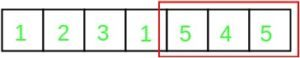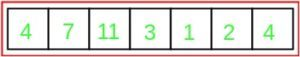# Find the smallest subarray having atleast one duplicate

Given an array arr of N elements, the task is to find the length of the smallest subarray of the given array that contains at least one duplicate element. A subarray is formed from consecutive elements of an array. If no such array exists, print “-1”.

Examples:

```Input: arr = {1, 2, 3, 1, 5, 4, 5}
Output: 3
Explanation:Input: arr = {4, 7, 11, 3, 1, 2, 4}
Output: 7
Explanation:```

## Recommended: Please try your approach on {IDE} first, before moving on to the solution.

Naive Approach:

• The trick is to find all pairs of two elements with equal value. Since these two elements have equal value, the subarray enclosing them would have at least a single duplicate and will be one of the candidates for the answer.
• A simple solution is to use two nested loops to find every pair of elements.If the two elements are equal then update the maximum length obtained so far.

Below is the implementation of the above approach:

## C++

 `// C++ program to find ` `// the smallest subarray having ` `// atleast one duplicate ` `#include ` `using` `namespace` `std; ` ` `  `// Function to calculate ` `// SubArray Length ` `int` `subArrayLength(``int` `arr[], ``int` `n) ` `{ ` ` `  `    ``int` `minLen = INT_MAX; ` ` `  `    ``for` `(``int` `i = 1; i < n; i++) { ` `        ``for` `(``int` `j = 0; j < i; j++) { ` `            ``// If the two elements are equal, ` `            ``// then the subarray arr[i..j] ` `            ``// will definitely have a duplicate ` `            ``if` `(arr[i] == arr[j]) { ` `                ``// Update the minimum length ` `                ``// obtained so far ` `                ``minLen = min(minLen, i - j + 1); ` `            ``} ` `        ``} ` `    ``} ` `    ``if` `(minLen == INT_MAX) { ` `        ``return` `-1; ` `    ``} ` ` `  `    ``return` `minLen; ` `} ` `// Driver Code ` `int` `main() ` `{ ` `    ``int` `n = 7; ` `    ``int` `arr[] = { 1, 2, 3, 1, 5, 4, 5 }; ` ` `  `    ``int` `ans = subArrayLength(arr, n); ` `    ``cout << ans << ``'\n'``; ` ` `  `    ``return` `0; ` `} `

## Java

 `// Java program to find  ` `// the smallest subarray having  ` `// atleast one duplicate ` ` `  `class` `GFG ` `{ ` `     `  `    ``final` `static` `int` `INT_MAX = Integer.MAX_VALUE; ` `     `  `    ``// Function to calculate  ` `    ``// SubArray Length  ` `    ``static` `int` `subArrayLength(``int` `arr[], ``int` `n)  ` `    ``{  ` `     `  `        ``int` `minLen = INT_MAX;  ` `     `  `        ``for` `(``int` `i = ``1``; i < n; i++)  ` `        ``{  ` `            ``for` `(``int` `j = ``0``; j < i; j++)  ` `            ``{  ` `                ``// If the two elements are equal,  ` `                ``// then the subarray arr[i..j]  ` `                ``// will definitely have a duplicate  ` `                ``if` `(arr[i] == arr[j])  ` `                ``{  ` `                    ``// Update the minimum length  ` `                    ``// obtained so far  ` `                    ``minLen = Math.min(minLen, i - j + ``1``);  ` `                ``}  ` `            ``}  ` `        ``}  ` `        ``if` `(minLen == INT_MAX) ` `        ``{  ` `            ``return` `-``1``;  ` `        ``}  ` `     `  `        ``return` `minLen;  ` `    ``}  ` `     `  `    ``// Driver Code  ` `    ``public` `static` `void` `main(String[] args) ` `    ``{  ` `        ``int` `n = ``7``;  ` `        ``int` `arr[] = { ``1``, ``2``, ``3``, ``1``, ``5``, ``4``, ``5` `};  ` `     `  `        ``int` `ans = subArrayLength(arr, n);  ` `        ``System.out.println(ans);  ` `         `  `    ``}  ` `} ` ` `  `// This code is contributed by AnkitRai01 `

## Python

 `# Python program for above approach ` `n ``=` `7` `arr ``=` `[``1``, ``2``, ``3``, ``1``, ``5``, ``4``, ``5``] ` `minLen ``=` `n ``+` `1` ` `  `for` `i ``in` `range``(``1``, n): ` `    ``for` `j ``in` `range``(``0``, i): ` `        ``if` `arr[i]``=``=` `arr[j]: ` `            ``minLen ``=` `min``(minLen, i``-``j ``+` `1``) ` ` `  `if` `minLen ``=``=` `n ``+` `1``: ` `       ``print``(``"-1"``) ` `else``: ` `       ``print``(minLen) `

## C#

 `// C# program to find  ` `// the smallest subarray having  ` `// atleast one duplicate ` `using` `System; ` ` `  `class` `GFG ` `{ ` `     `  `    ``static` `int` `INT_MAX = ``int``.MaxValue; ` `     `  `    ``// Function to calculate  ` `    ``// SubArray Length  ` `    ``static` `int` `subArrayLength(``int` `[]arr, ``int` `n)  ` `    ``{  ` `     `  `        ``int` `minLen = INT_MAX;  ` `     `  `        ``for` `(``int` `i = 1; i < n; i++)  ` `        ``{  ` `            ``for` `(``int` `j = 0; j < i; j++)  ` `            ``{  ` `                ``// If the two elements are equal,  ` `                ``// then the subarray arr[i..j]  ` `                ``// will definitely have a duplicate  ` `                ``if` `(arr[i] == arr[j])  ` `                ``{  ` `                    ``// Update the minimum length  ` `                    ``// obtained so far  ` `                    ``minLen = Math.Min(minLen, i - j + 1);  ` `                ``}  ` `            ``}  ` `        ``}  ` `        ``if` `(minLen == INT_MAX) ` `        ``{  ` `            ``return` `-1;  ` `        ``}  ` `     `  `        ``return` `minLen;  ` `    ``}  ` `     `  `    ``// Driver Code  ` `    ``public` `static` `void` `Main() ` `    ``{  ` `        ``int` `n = 7;  ` `        ``int` `[]arr = { 1, 2, 3, 1, 5, 4, 5 };  ` `     `  `        ``int` `ans = subArrayLength(arr, n);  ` `        ``Console.WriteLine(ans);  ` `         `  `    ``}  ` `} ` ` `  `// This code is contributed by AnkitRai01 `

Output:

```3
```

Time Complexity: O(N2)

Efficient Approach:

This problem can be solved in O(N) time and O(N) Auxillary space using the idea of hashing technique. The idea is to iterate through each element of the array in a linear way and for each element, find its last occurrence using a hashmap and then update the value of min length using the difference of the last occurrence and the current index. Also, update the value of the last occurrence of the element by the value of the current index.

Below is the implementation of the above approach:

## C++

 `// C++ program to find ` `// the smallest subarray having ` `// atleast one duplicate ` `#include ` `using` `namespace` `std; ` ` `  `// Function to calculate ` `// SubArray Length ` `int` `subArrayLength(``int` `arr[], ``int` `n) ` `{ ` ` `  `    ``int` `minLen = INT_MAX; ` `    ``// Last stores the index of the last ` `    ``// occurrence of the corresponding value ` `    ``unordered_map<``int``, ``int``> last; ` ` `  `    ``for` `(``int` `i = 0; i < n; i++) { ` `        ``// If the element has already occurred ` `        ``if` `(last[arr[i]] != 0) { ` `            ``minLen = min(minLen, i - last[arr[i]] + 2); ` `        ``} ` `        ``last[arr[i]] = i + 1; ` `    ``} ` `    ``if` `(minLen == INT_MAX) { ` `        ``return` `-1; ` `    ``} ` ` `  `    ``return` `minLen; ` `} ` ` `  `// Driver Code ` `int` `main() ` `{ ` `    ``int` `n = 7; ` `    ``int` `arr[] = { 1, 2, 3, 1, 5, 4, 5 }; ` ` `  `    ``int` `ans = subArrayLength(arr, n); ` `    ``cout << ans << ``'\n'``; ` ` `  `    ``return` `0; ` `} `

## Java

 `// Java program to find ` `// the smallest subarray having ` `// atleast one duplicate ` `import` `java.util.*; ` ` `  `class` `GFG  ` `{ ` ` `  `    ``// Function to calculate ` `    ``// SubArray Length ` `    ``static` `int` `subArrayLength(``int` `arr[], ``int` `n) ` `    ``{ ` ` `  `        ``int` `minLen = Integer.MAX_VALUE; ` `         `  `        ``// Last stores the index of the last ` `        ``// occurrence of the corresponding value ` `        ``HashMap last = ``new` `HashMap(); ` ` `  `        ``for` `(``int` `i = ``0``; i < n; i++)  ` `        ``{ ` `            ``// If the element has already occurred ` `            ``if` `(last.containsKey(arr[i]) && last.get(arr[i]) != ``0``)  ` `            ``{ ` `                ``minLen = Math.min(minLen, i - last.get(arr[i]) + ``2``); ` `            ``} ` `            ``last.put(arr[i], i + ``1``); ` `        ``} ` `        ``if` `(minLen == Integer.MAX_VALUE) ` `        ``{ ` `            ``return` `-``1``; ` `        ``} ` ` `  `        ``return` `minLen; ` `    ``} ` ` `  `    ``// Driver Code ` `    ``public` `static` `void` `main(String[] args)  ` `    ``{ ` `        ``int` `n = ``7``; ` `        ``int` `arr[] = { ``1``, ``2``, ``3``, ``1``, ``5``, ``4``, ``5` `}; ` ` `  `        ``int` `ans = subArrayLength(arr, n); ` `        ``System.out.print(ans); ` `    ``} ` `} ` ` `  `// This code is contributed by 29AjayKumar `

## Python

 `# Python program for above approach ` ` `  `n ``=` `7` `arr ``=` `[``1``, ``2``, ``3``, ``1``, ``5``, ``4``, ``5``] ` ` `  `last ``=` `dict``() ` ` `  `minLen ``=` `n ``+` `1` ` `  `for` `i ``in` `range``(``0``, n): ` `    ``if` `arr[i] ``in` `last: ` `        ``minLen ``=` `min``(minLen, i``-``last[arr[i]]``+``2``) ` ` `  `    ``last[arr[i]]``=` `i ``+` `1`     ` `  ` `  `if` `minLen ``=``=` `n ``+` `1``: ` `       ``print``(``"-1"``) ` `else``: ` `       ``print``(minLen) `

## C#

 `// C# program to find ` `// the smallest subarray having ` `// atleast one duplicate ` `using` `System; ` `using` `System.Collections.Generic; ` ` `  `class` `GFG  ` `{ ` ` `  `    ``// Function to calculate ` `    ``// SubArray Length ` `    ``static` `int` `subArrayLength(``int` `[]arr, ``int` `n) ` `    ``{ ` ` `  `        ``int` `minLen = ``int``.MaxValue; ` `         `  `        ``// Last stores the index of the last ` `        ``// occurrence of the corresponding value ` `        ``Dictionary<``int``, ``int``> last = ``new` `Dictionary<``int``, ``int``>(); ` ` `  `        ``for` `(``int` `i = 0; i < n; i++)  ` `        ``{ ` `            ``// If the element has already occurred ` `            ``if` `(last.ContainsKey(arr[i]) && last[arr[i]] != 0)  ` `            ``{ ` `                ``minLen = Math.Min(minLen, i - last[arr[i]] + 2); ` `            ``} ` `            ``if``(last.ContainsKey(arr[i])) ` `                ``last[arr[i]] = i + 1; ` `            ``else` `                ``last.Add(arr[i], i + 1); ` `        ``} ` `        ``if` `(minLen == ``int``.MaxValue) ` `        ``{ ` `            ``return` `-1; ` `        ``} ` ` `  `        ``return` `minLen; ` `    ``} ` ` `  `    ``// Driver Code ` `    ``public` `static` `void` `Main(String[] args)  ` `    ``{ ` `        ``int` `n = 7; ` `        ``int` `[]arr = { 1, 2, 3, 1, 5, 4, 5 }; ` ` `  `        ``int` `ans = subArrayLength(arr, n); ` `        ``Console.Write(ans); ` `    ``} ` `} ` ` `  `// This code is contributed by PrinciRaj1992 `

Output:

```3
```

Time Complexity: O(N), where N is size of array

My Personal Notes arrow_drop_upCheck out this Author's contributed articles.

If you like GeeksforGeeks and would like to contribute, you can also write an article using contribute.geeksforgeeks.org or mail your article to contribute@geeksforgeeks.org. See your article appearing on the GeeksforGeeks main page and help other Geeks.

Please Improve this article if you find anything incorrect by clicking on the "Improve Article" button below.